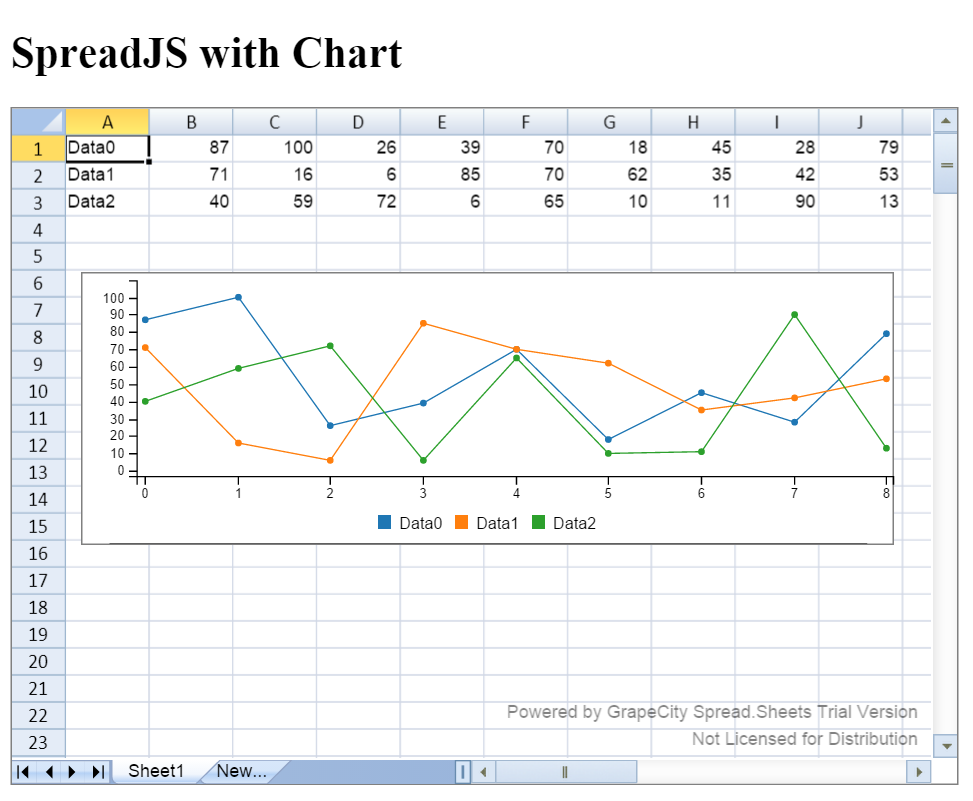SpreadJS is a useful tool for adding the power of Spread to webpages using JavaScript. Being able to easily display data in a spreadsheet without having to create html tables by hand is useful for web developers who simply want to convey information. SpreadJS has the concept of Sparklines, which are small graphs contained within cells. While this is useful, it might also be desirable to create large graphs that don’t depend on being inside of a cell. To do this, we can use a Custom Floating Object to wrap a third-party chart into SpreadJS. In this blog, I will show you how to insert a D3-based chart inside of SpreadJS. To start off, I needed some sample data to display in the chart, so I created a function that simply generates new data every time the page loads:

``````var data = (function () {
var chartData = [];
for (var i = 0; i < 3; i++) {
var columnData = [];
columnData.push("Data" + i);
for (var j = 0; j < 10; j++) {
columnData.push( Math.round(Math.random() * 100));
}
chartData.push(columnData);
}
return chartData;
})();
``````

Next, I created a spread component on the page and placed the data in it:

``````var spread = new GcSpread.Sheets.Spread(\$("#ss").get(0));
activeSheet.isPaintSuspended(true);
activeSheet.setArray(0, 0, data);
activeSheet.isPaintSuspended(false);
``````

To add the chart, we first have to create a floating object that will contain it, and add it to the sheet:

``````var floatChart = new GcSpread.Sheets.CustomFloatingObject("chart", 10, 100, 600, 200);
floatChart.Content(\$("<div style="background: white; border: 1px solid gray;"></div>"));
``````

Now that the container is there, we have to put a chart in it. To do this, we have to bind the CustomFloatingObjectLoaded event to a new handler function:

``````activeSheet.bind(GcSpread.Sheets.Events.CustomFloatingObjectLoaded, function (sender, args) {
var chartContainer = \$(args.element);
chartContainer.attr("id", "chart");
chart = c3.generate({
data: {
columns: args.sheet.getArray(0,0,3,10)
}
});
});
``````

With the c3 library, the type of chart defaults as a line chart. To change the type of chart, simply specify the “types” property of data for each series. More information on using c3 can be found here: http://c3js.org/gettingstarted.html. To ensure that the chart changes size with the floating object, we can bind a function to the FloatingObjectChanged event:

``````activeSheet.bind(GcSpread.Sheets.Events.FloatingObjectChanged, function (sender, args) {
if (args.propertyName === "width" || args.propertyName === "height") {
chart.resize({ width: args.floatingObject.width(), height: args.floatingObject.height() });
}
});
``````

Lastly, if that data changes, we can have those changes reflected in the chart by binding a function to the ValueChanged event:

``````activeSheet.bind(GcSpread.Sheets.Events.ValueChanged, function (sender, args) {
chart = c3.generate({
data: {
columns: args.sheet.getArray(0, 0, 3, 10)
}
});
});
``````

When these lines of code are added as script for a page, we can see that there is a Spread component with a nice graph that can be moved around and resized. It also reflects the data in the sheet, so we won’t have to reload the page with new data every time we want to change the graph. The download for this sample can be found at the bottom of this blog.This is the completed webpage with SpreadJS SpreadJS ChartsGrapeCity

GrapeCity Developer Tools